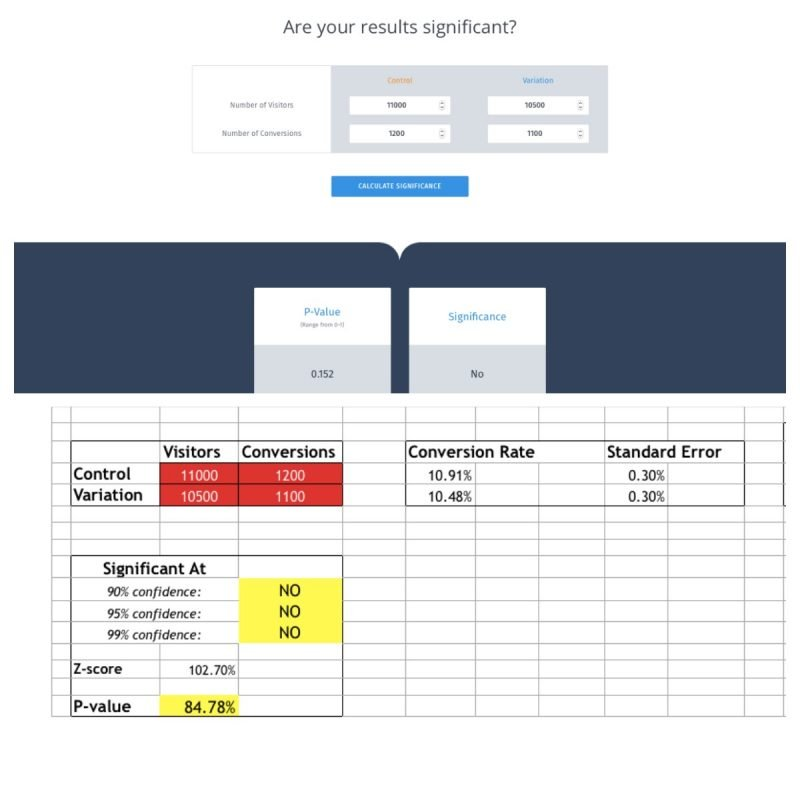This website works best with JavaScript enabled. Learn how to enable JavaScript.

# How VWO Calculates Statistical Significance

Last updated

You can use our Test Duration Calculator to find out the statistical significance required for your business needs. Alternatively, you can download this excel spreadsheet to calculate the data yourself.

Note that the Test Duration Calculator and the excel spreadsheet uses Frequentist Method to calculate data. However, the statistical significance used in Frequentist Method does not apply to A/B and Split tests in VWO, which are based on Bayesian statistics.

You may observe that the ‘significance value’ is different in the calculator and excel spreadsheet. The reason is that the excel spreadsheet shows the exact ‘p-value’, while the significance calculator will give ‘p-value’ between 1-p, if the p-value comes out to be more than 0.5. If the p-value is less than 0.5, both the duration calculator as well as excel sheet will show the same value.

#### Use Case 2: If the p-value is different in both calculatorsRefer to our KB article on Bayesian statistics and the mathematics behind calculating SmartStats. For revenue goals, download this excel file to understand the statistical significance.

#### Was this article helpful?

Thanks for your feedback!
Please email us at support@vwo.com to tell us what you were looking for. We'll send the answer to your inbox.